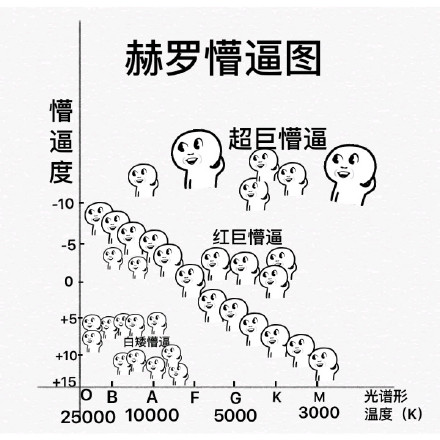# 【回炉重造】超详细的Java运算符修炼手册（优秀程序员不得不知道的运算技巧）

~（按位取反）

1.不用临时变量交换两个数

2.在成对数中找单独数

3.在单独数中找成对数

“与运算”的特殊用途：

“或运算”特殊作用：

++（自增）- -（自减）

+ - * / （加减乘除）

%（取余）

取余常用于判断奇偶：

==，!=，>，<，>=，<=

<< 带符号左移

>>带符号右移

>>> 无符号右移

=、+=、-=、*=、/=、%=、&=、^=、|=、<<=、>>=

# 算数运算符

## ~（按位取反）

### 二进制存放形式、补码、反码

• 二进制数在内存中是以补码的形式存放的；补码首位是符号位，0表示此数为正数，1表示此数为负数

9的二进制是1001，它的补码就是01001，第一位0表示正数

• 正数、负数的补码和反码是什么？

### 按位取反 "~" 运算符原理

• 按位取反（包括符号位）得到10110

• 补码转原码：11010
• 二进制转十进制：-10（记住头一位是表示正负）

• 按位取反（包括符号位）得到01001

• 补码转原码：01001
• 二进制转十进制：9（记住头一位是表示正负）

    public static void main(String[] args) {
for(int i=0;i<1000;i++){
System.out.println("~"+i+"按位取反="+~i);
}
}

得出结果：

~0按位取反= -1
~1按位取反= -2
~2按位取反= -3
~3按位取反= -4
~4按位取反= -5

.......省略
~995按位取反= -996
~996按位取反= -997
~997按位取反= -998
~998按位取反= -999
~999按位取反= -1000

负数

    public static void main(String[] args) {
for(int i=0;i>-1000;i--){
System.out.println("~"+i+"按位取反="+~i);
}
}


得出结果：

~0按位取反= -1
~-1按位取反= 0
~-2按位取反= 1
~-3按位取反= 2
~-4按位取反= 3

......省略

~-995按位取反= 994
~-996按位取反= 995
~-997按位取反= 996
~-998按位取反= 997
~-999按位取反= 998

得出规律结论

## 位异或运算（^）

    public static void main(String[] args){
//19二进制：0001 0011
//20二进制：0001 0100
//异或结果: 0000 0111
System.out.println(19^20);//7
}

    public static void main(String[] args){
//20二进制：0001 0100
//20二进制：0001 0100
//异或结果: 0000 0000
System.out.println(20^20);//0
}


### 1.不用临时变量交换两个数

    public static void main(String[] args){
int a=4,b=3;
int temp = a;
a = b;
b = temp;
System.out.println("a="+a+",b="+b);//a=3,b=4
}

    public static void main(String[] args){
int a=4,b=3;
a=a^b;
b=a^b;
a=a^b;
System.out.println("a="+a+",b="+b);//a=3,b=4
}

### 2.在成对数中找单独数

    public static void main(String[] args){
int[] array = {2,3,4,4,3,5,6,6,5};
int v = 0;
for (int i = 0;i < array.length;i++) {
v ^= array[i];
}
System.out.println("只出现一次的数是:" + v);//只出现一次的数是:2
}

### 3.在单独数中找成对数

    public static void main(String[] args){
int[] array = {1,2,3,4,5,6,7,8,9,10,10};
int v = 0,k = 0;
for (int i = 0;i < array.length;i++) {
v ^= array[i];
k ^= i<array.length-1?array[i]:0;
}
System.out.println("出现两次的数是:" + (v^k));//出现两次的数是:10
}

## 位与运算符（&）

129转换成二进制就是10000001，128转换成二进制就是10000000。从高位开始比较得到，得到10000000，即128。

### “与运算”的特殊用途：

（1）清零。如果想将一个单元清零，即使其全部二进制位为0，只要与一个各位都为零的数值相与，结果为零。

（2）取一个数中指定位

取X的低4位，用 X & 0000 1111 = 0000 1110 即可得到；

还可用来取X的2、4、6位。

## 按位或运算符（|）

即 ：参加运算的两个对象只要有一个为1，其值为1。

### “或运算”特殊作用：

（1）常用来对一个数据的某些位置换为1。

## ++（自增）- -（自减）

++ --运算符的使用:

• （i++，i--）运算符在操作数后面，先赋值，再运算
• （++i， --i）运算符在操作数前面，先运算，再赋值

// 示例一
int i = 1;
i = i++;
System.out.println(i);
// 输出结果 1
------分割-----------------------
// 示例二
int i = 1;
i = ++i;
System.out.println(i);
// 输出结果 2

示例二，++i,++在左边，所以是先自增再赋值。所以++i是先将 "i" 加1这时 "i" == 2，再赋值给 "i"所以打印为 2

int x = 3;

int y = x++ + ++x + x * 10;

byte b = 5;

b++;

b = b +1;

b是byte类型的数,在参与运算时会自动类型提升为int数,因此b + 1的结果是个int数,int数要赋值给byte数,会报错:损失精度。

b++;

## + - * / （加减乘除）

java中的加减乘除与平时生活中运用的数学加减乘除误差。这里不做过多解释，看代码；

    public static void main(String[] args) {
System.out.println(3+3); //结果：6
System.out.println(3-3); //结果：0
System.out.println(3*3); //结果：9
System.out.println(3/3); //结果：1
}

## %（取余）

### 取余常用于判断奇偶：

    public static void main(String[] args) {
int n = 123;
if(n%2==0){
System.out.println("偶数");
}else{
System.out.println("基数");
}
}

### 关于-10%-3=-1的问题

    public static void main(String[] args) {
int a=-10,b=-3;
System.out.print(a%b);
}

答案出乎意料的是 -1，什么鬼？

    public static void main(String[] args) {
int a=-10,b=3;
System.out.print(a%b);
}

    public static void main(String[] args) {
int a=10,b=-3;
System.out.print(a%b);
}


• 余数是指整数除法中被除数未被除尽部分。
• 余数和除数的差的绝对值要小于除数的绝对值（适用于实数域）；
• 所以从定义上来说，负数除以负数，余数可以是负数。
• 在java中的定义就是遵循上面定义。

## 三目运算（ 三元运算）

• 布尔类型表达式结果是 true，三元运算符整体结果为结果1，赋值给变量。
• 布尔类型表达式结果是 false，三元运算符整体结果为结果2，赋值给变量。

### 运用案例

public static void main(String[] args) {
int i = (1==2 ? 100 : 200);
System.out.println(i);//200
int j = (3<=4 ? 500 : 600);
System.out.println(j);//500
}

    public static void main(String[] args) {
int a=1,b=2;
//谁大输出谁
if(a>b){
System.out.print(a);
}else{
System.out.print(b);
}
}

    public static void main(String[] args) {
int a=1,b=2;
//谁大输出谁
System.out.println(a>b?a:b);
}

### 三元运算符和if_else效率问题

• 程序运行时，处理器会通过并行运算而加速运行，当遇到选择支时则会停下判断 (例如高速行驶的大卡车遇到了分岔路）if-else 是先赋值再运算，为了节省时间，分支预测会先猜测运行 if 还是 else 并继续运行 (默认是if)，若猜对则因并行运算而节省时间，若猜错则因消除运算而耗费时间。 (卡车直接冲向一边康康可不可以走通,如果可以则继续走，如果不可以则回头走另一条路)(成本是回到分岔处的时间）
• 三目 是先运算再赋值，遇到选择支时停止并行并判断条件。(在分岔处停下康地图) (成本是重新加速需要的时间)，在多数情况下，运算结果为0与为1的可能相近，分支预测&并行运算 会比三目耗费更多的时间，所以应更多的使用三目。在一些情况下，运算结果大多为0或大多为1（80+%），这时 分支预测&并行运算 的损耗远小于三目，所以应选择 if-else

# 关系运算符

## ==，!=，>，<，>=，<=

==：比较符号两边数据是否相等，相等结果是true。

!=：不等于符号 ，如果符号两边的数据不相等，结果是true。

>：比较符号左边的数据是否大于右边的数据，如果大于结果是true。

<：比较符号左边的数据是否小于右边的数据，如果小于结果是true。

>=：比较符号左边的数据是否大于或者等于右边的数据，如果小于结果是true。

<=：比较符号左边的数据是否小于或者等于右边的数据，如果小于结果是true。

• 比较运算符，是两个数据之间进行比较的运算，运算结果都是布尔值 true 或者 false 。
public static void main(String[] args) {
System.out.println(1==1);//true
System.out.println(1<2);//true
System.out.println(3>4);//false
System.out.println(3<=4);//true
System.out.println(3>=4);//false
System.out.println(3!=4);//true
}

# 逻辑运算符

## 与（&&）、（&）

&&使用

• 两边都是true，结果是true
• 一边是false，结果是false
• 短路特点：符号左边是false，右边不再运算

&则是没有短路特性

## 或（||）、（|）

• 两边都是false，结果是false
• 一边是true，结果是true
• 短路特点： 符号左边是true，右边不再运算

|则是没有短路特性

! true 结果是false
! false结果是true

# 位移运算符

## << 带符号左移

    public static void main(String[] args){
int a=2;
System.out.print("a 移位的结果是"+(a<<2));//a 移位的结果是8
}

## >>带符号右移

    public static void main(String[] args){
int a=8;
System.out.print("a 移位的结果是"+(a>>2));//a 移位的结果是2
}

## >>> 无符号右移

    public static void main(String[] args){
int a=-8;
System.out.print("a 移位的结果是"+ (a>>>2));//a 移位的结果是1073741822
}（不理解补码向最上看，或者去看目录，上面有提到）

1 00000000 00000000 00000000 00001000 （-8的二进制，第一位符号位） 1 11111111 11111111 11111111 11111000 （内部补码存放形式） 0 00111111 11111111 11111111 11111110 （进行位移运算后，此时符号位为0是正数，正数的补码转原码就是其本身）

00111111 11111111 11111111 11111110 也就是十进制的 1073741822

# 赋值运算符

## =、+=、-=、*=、/=、%=、&=、^=、|=、<<=、>>=

• 赋值运算符，就是将符号右边的值，赋给左边的变量。

public static void main(String[] args){
int i = 5;
i+=5;//计算方式 i=i+5 变量i先加5，再赋值变量i
System.out.println(i); //输出结果是10
}©️2019 CSDN 皮肤主题: 数字20 设计师: CSDN官方博客